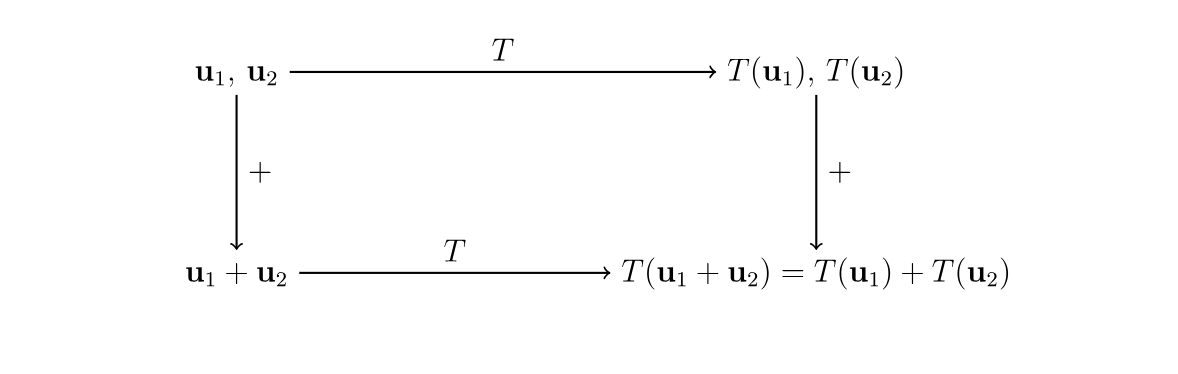# Linear Transformation

## What is a Linear Transformation?

A Linear Transformation, also known as a linear map, is a mapping of a function between two modules that preserves the operations of addition and scalar multiplication. In short, it is the transformation of a function T

from the vector space

U, also called the domain, to the vector space V, also called the codomain. ( T : U → V ) The linear transformation has two additional properties:
1. T(u1+u2)=T(u1)+T(u2) for all u1,u2∈U
2. T(αu)=αT(u)T(αu)=αT(u) for all u∈U and all α∈C
In essence, these properties mean every vector space property derives from vector addition and scalar multiplication. These properties apply to every property of linear transformations.

## How does a Linear Transformation work?

The diagram below exemplifies a linear transformation that is additive, meaning the functions are added together. Beginning in the upper left-hand corner, the transformation follows the two pathways, as diagrammed by the arrows. The two produced expressions of the linear transformation are always equal. Notice that while T is the name of the function, T(u), is the name of the output of the transformation.Image Credit: https://www.linear.ups.edu

## Applications of Linear Transformations

Linear transformations are used in both abstract mathematics, as well as computer science. Linear transformations within calculus are used as way of tracking change, also known as derivatives.

### Linear Transformations and Machine Learning

Linear transformations are often used in machine learning applications. They are useful in the modeling of 2D and 3D animation, where an objects size and shape needs to be transformed from one viewing angle to the next. An object can be rotated and scaled within a space using a type of linear transformations known as geometric transformations, as well as applying transformation matrices.